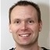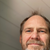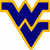0

# Looking for way to reset the Integrate(operand) function

Dan Gray 7 years ago in IQANdesign updated by Joe 5 years ago
Topic collaboratorsI'm trying to use the Integrate(operand) function in a math channel and I would like to be able to reset the function under some circumstances. The only way I can see to do this is to use several lines of qcode. For example:

A:= DerivativeOf(Positon)
B:= Reset
C:= Integrate(Abs(A)) //use absolute value to get total distance traveled
if C = 0 then
D1:=0 //D1 is the starting point
endif
D2:= C //D2 is the end point
D:= D2 - D1 //D is the distance traveled between resets
if B = true then
D1:= C
endif
Result:= D

Is there an easier way to reset the Integrate(operand) function? Perhaps the function could have one or two added arguments, for example Integrate(Operand1, Operand2, Operand3) where Operand 1 is the part to be integrated, Operand 2 is the reset channel, and Operand3 is the reset value?

Thanks,

DanGOOD, I'M SATISFIED

Thank you for the help.

Satisfaction mark by Dan Gray 7 years agoHi Dan,

The Integrate() function can be reset if the channel where you use it is placed in a function group that you disable.

Another approach is to use Qcode to make an alternative integrate function using a static variable. A few more steps, but you would be able to reset it within the channel.

The topic was posted as a private topic, would you mind if I move this to the public forum discussion?Hi. Thanks for the reply. I haven't used static variables yet, so I'll have to give it a try. Yes, you can move this to a public forum.